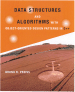Data Structures and Algorithms with Object-Oriented Design Patterns in C++## Multiplication Method

A very simple variation on the middle-square method that alleviates its deficiencies is the so-called, multiplication hashing method  . Instead of multiplying the key x by itself, we multiply the key by a carefully chosen constant a, and then extract the middle k bits from the result. In this case, the hashing function is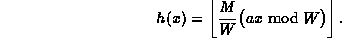What is a suitable choice for the constant a? If we want to avoid the problems that the middle-square method encounters with keys having a large number of leading or trailing zeroes, then we should choose an a that has neither leading nor trailing zeroes.

Furthermore, if we choose an a that is relatively primeto W, then there exists another number a' such that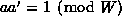. In other words, a' is the inverse  of a modulo W, since the product of a and its inverse is one. Such a number has the nice property that if we take a key x, and multiply it by a to get ax, we can recover the original key by multiplying the product again by a', since axa'=aa'x=1x.

There are many possible constants which the desired properties. One possibility which is suited for 32-bit arithmetic (i.e.,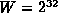) is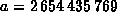. The binary representation of a is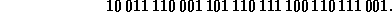This number has neither many leading nor trailing zeroes. Also, this value of a andare relatively prime and the inverse of a modulo W is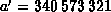.

The following code fragment illustrates the multiplication method of hashing:

```unsigned int const k = 10; // M==1024
unsigned int const w = bitsizeof (unsigned int);
unsigned int const a = 2654435769U;

unsigned int h (unsigned int x)
{ return (x * a) >> (w - k); }```
Note, this implementation assumes that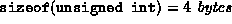. I.e., the natural word size of the machine is 32 bits. The code is a simple modification of the middle-square version. Nevertheless, the running time remains O(1).Copyright © 1997 by Bruno R. Preiss, P.Eng. All rights reserved.